Mirrors

Flat

For a flat mirror, the angle of incidence is equal to the angle of reflection: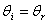This is called the law of reflection.

The image forms by reflection of light from the flat mirror is located behind the mirror, as in the picture below.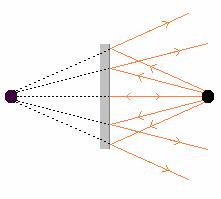The object distance is equal to the image distance, so the magnification equals one:The image height is equal to the object height.

Concave

For concave mirrors, the law of reflection can be applied to find the location of an object. When the rays of light striking a concave mirror are parallel, they all converge at a point, as in the picture below. This point is called the focal point of the mirror.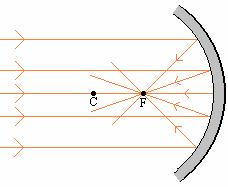The point C is the center of curvature of the lens. The distance of the focal point to the mirror is called the focal length, f, of the mirror. The distance of the center of curvature to the mirror is called the radius of curvature, R, of the mirror.

The rules for drawing ray diagrams for mirrors are:

1.     A ray from the top of the object that strikes the mirror parallel to the axis of the mirror is reflected through the focal point.

2.     A ray from the top of the object that passes through the focal point is reflected parallel to the axis of the mirror.

3.     A ray from the top of the object through the center of curvature C is reflected back on itself.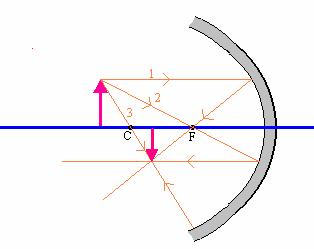It can be shown (and will not be shown here) that the focal length (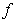), the object distance (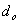) and the image distance (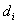) are related by the following equation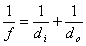with the following set of rules:

1.is positive if the object is in front of the mirror and negative if it is behind the mirror.

2.is positive if the image is in front of the mirror and negative if it is behind the mirror.

3. The focal length,, is positive for a concave mirror and negative for a convex mirror.

The magnification is defined as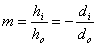Convex

For convex mirrors, the law of reflection can be applied to find the location of an object. When the rays of light striking a convex mirror are parallel, they all appear to emerge from a point, as in the picture below. This point is called the focal point of the mirror.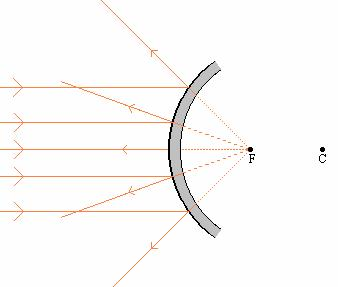The point C is the center of curvature of the lens. The distance of the focal point to the mirror is called the focal length, f, of the mirror. The distance of the center of curvature to the mirror is called the radius of curvature, R, of the mirror. The focal length and the radius of curvature are related by: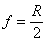The rules for drawing ray diagrams are the same as for a concave mirror are:

1.     A ray from the top of the object that strikes the mirror parallel to the axis of the mirror is reflected through the focal point.

2.     A ray from the top of the object that passes through the focal point is reflected parallel to the axis of the mirror.

3.     A ray from the top of the object through the center of curvature C is reflected back on itself.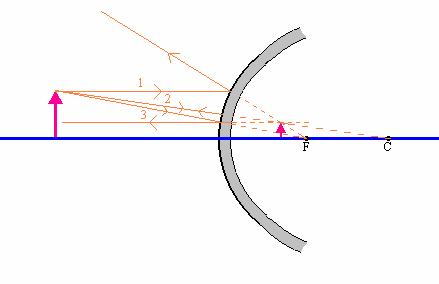It can be shown (and will not be shown here) that the focal length (), the object distance () and the image distance () are related by the following equationwith the following set of rules:

1.is positive if the object is in front of the mirror and negative if it is behind the mirror.

2.is positive if the image is in front of the mirror and negative if it is behind the mirror.

3. The focal length,, is positive for a concave mirror and negative for a convex mirror.

The magnification is defined as